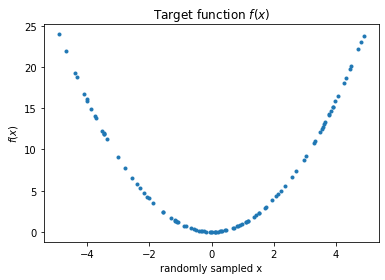# How to Develop a 1D Generative Adversarial Network From Scratch in PyTorch (Part 1)

## Goal¶

This post is inspired by the blog "Machine Learning Mastery - How to Develop a 1D Generative Adversarial Network From Scratch in Keras" written by Jason Brownlee, PhD. But to learn step-by-step, I will describe the same concept with PyTorch.

This post will cover the followings:

Part 1:

• Select a One-Dimensional Function
• Define a Discriminator Model

Reference

## Libraries¶

In :
import pandas as pd
import numpy as np
import matplotlib.pyplot as plt
%matplotlib inline

# PyTorch
import torch
from torch import nn
from torch import optim
from torchviz import make_dot


## Create a target 1-D function¶

In :
def f(x):
return x **2

In :
n = 100
sigma = 10
x = sigma * (np.random.random(size=n) - 0.5)
plt.plot(x, f(x), '.');
plt.title('Target function $f(x)$');
plt.xlabel('randomly sampled x');
plt.ylabel('$f(x)$');## Define a Discriminator Model¶

The definition of a discriminator model is that it will classify the input data into real or fake

In :
# Build a feed-forward network
model = nn.Sequential(nn.Linear(2, 25),
nn.ReLU(),
nn.Sigmoid()
)

# Loss
criterion = nn.CrossEntropyLoss()

# Optimizer

In :
# Visualize this neural network
x = torch.zeros(0, 2, dtype=torch.float, requires_grad=False)
out = model(x)
make_dot(out)

Out:

## Create real and fake samples¶

In :
def generate_samples(size=100, label='real'):
"""Generate samples with real or fake label
"""
x = np.random.randn(size, 1)
x2 = f(x)

y = np.ones((size, 1)) * (label == 'real')
return np.hstack([x, x2]), y


In :
X, y = generate_samples()

In :
x[:5]

Out:
array([[ 1.2483621 ,  1.55840793],
[ 0.57980381,  0.33617245],
[-0.06718955,  0.00451444],
[-1.95352245,  3.81624995],
[-1.14922801,  1.32072501]])
In :
y[:5]

Out:
array([[1.],
[1.],
[1.],
[1.],
[1.]])